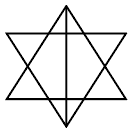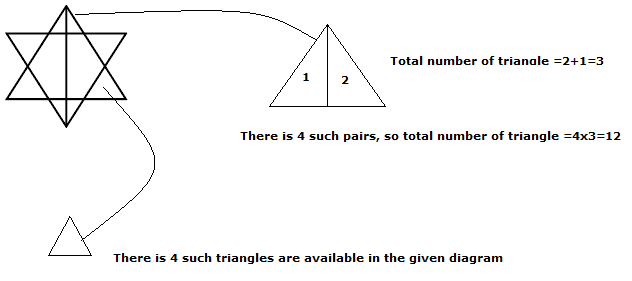Question 17

# How many triangles are there in the following figure ?Solution

As per the given question,The two large triangle in the centre divided by a vertical line.
Hence, the total number of triangle =12 + 4 +2=18

• Free SSC Study Material - 18000 Questions
• 230+ SSC previous papers with solutions PDF